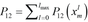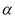Actomyosin Cycles Implemented in MUSICO Platform

The development of the MUSICO framework will yield a model of muscle contraction that accounts for the details of chemo-mechanical transduction and molecular dynamics. The availability of such a tool promises to increase our understanding of normal and abnormal muscle contractility beyond what is currently known. For example, the model of Piazzesi and Lombardi which assumes the periodicity of myosin binding sites on actin, implies that myosin heads could slew azimuthally to sites with an angular mismatch of up to 90°. However, azimuthal selection could be much tighter, leading to models with a lower fraction of bound heads under isometric conditions. The myosin binding models under development attempt to reveal several fundamental tenets of molecular scale contractility in order to achieve mechano-chemical coupling in leaving muscle cells:

A probabilistic formulation of crossbridge kinetics. The mathematical formulation of sliding filament system at the level of a single myosin head is given in terms of the state probability density function,          , i.e. the probability of the head being attached to the thin filament (actin) at an binding site displaced by distance    at time    (Eulerian formulation). The subscript  identifies the particular actomyosin state and runs from 1 to the total number of states     used in the crossbridge cycle. The myosin may be detached or bound to actin. For simplicity, in the mass action simulations presented here, we simplified the spatially distributed elasticity of the filaments into a (discrete) series elastic component and consider myosin binding to actin as in the inextensible filament model. Note that in the MUSICO simulations, myofilament and crossbridge compliances are treated explicitly as a function of position along the filaments. The strain dependent state transitions between myosin states are governed by conservation laws expressed as field equations. In vector form, this system of partial differential equations becomes,

where         is the state transition rate matrix. The matrix size,         , is defined by the number of states in the actomyosin cycle, and, in general, this matrix in three dimensions depends on the relative spatial position of unstrained myosin heads and their binding sites on actin,                      .  We consider here only the axial strain dependence (exclusively of   ). More detailed dewscriopin in shown here.

State Transition Rates. The general form of the state transition rates in models with a power stroke is formulated by T.L. Hill 1974 as the ratio of forward to backward rates that must satisfy Gibbs’ thermodynamic identity

in terms of the Gibbs energies of the initial and final states, including the elastic strain energy derived from the crossbridge tension. Here         is the equilibrium rate constant between states    and   , and each forward or backward rate constant          is composed of a strain-independent rate        which, in principle, is the rate observed in a solution-kinetic experiment under the same conditions, and an -dependent, i.e. axial strain-dependent, function which is equal to 1 when the molecules are not tethered.

This general description is applicable to different actomyosin cycles that differ in number of states. So far in MUSICO platform (stochastic and probabilistic formulations)  are implemented: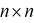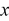Stochastic formulation of crossbridge kinetics. In the stochastic model, we employed the standard Metropolis algorithm where a kinetic transition in time step     is implemented when a random number in (0, 1) lies in the range (0,      ), where     is the first-order transition rate constant. This algorithm generates a Markov process if                 , so that at most one transition occurs per Monte Carlo time step in a single subsystem, here considered as two CFCs on one actin filament for TnI transitions or one myosin filament and its associated actin filaments for crossbridge kinetics, so interference between multiple transitions within a single subsystem is avoided, and between the systems negligibly small. Thus,      must be less than the inverse of the fastest rate constant of the system,         , and in practice                       was required to achieve satisfactory statistics.

The coupling between the actomyosin cycle and thin filament regulatory kinetics requires two sets of Monte Carlo drawings, and the overall numerical procedure within each time step includes: (1) the first set of  drawings defining transitions of TnI-actin states; (2)  calculation of chain configuration with updated TnI-actin states and myosin bound states from the previous time step; (3) a second drawing defining the changes on actomyosin states regulated by the CFC; and (4) calculation of the mechanical equilibrium with external forces and constraints. The last step includes an iterative procedure to account for nonlinear elasticities included in the mechanical system, for example nonlinear elasticity of titin.

For each TnI or crossbridge transitions we use one Monte Carlo drawing to define whether the TnI or crossbridge remains in its current state or it will change its state into one of the possible states within the current time step     . For each TnI or crossbridge the probability, in the range from 0 to 1, is divided into probability bins,       , in a specified order, including the set of probability bins associated with a TnI or crossbridge changing state and a bin associated with the probability of remaining in the current state. Depending in which bin the drawn random number falls, the fate of a particular TnI or crossbridge is defined and set for the following time step.

In a half sarcomere each myosin half-filament interacts with six actin filaments, but because of symmetry, each half of myosin filament effectively interacts with two actin filaments. The interactions between myosin heads (crossbridges) and myosin binding sites on actin in the 3-D sarcomere lattice are as described in (Mijailovich et al., 2016). Furthermore, each actin filament can be viewed as a double helix where each strand is associated with on CFC. We consider here that one CFC subsystem consists of one CFC and one actin strand.  Because each TmTn unit covers 7 actin monomers, the number of TnI-actin binding sites per strand is equal to one seventh of the number of actin monomers per strand (Mijailovich et al., 2012a).

Modulation of TnI-actin transitions by bound myosins. Interactions between TnI and actin are defined by two states and the transition between these states is defined by the equilibrium state transition constant                                       where         is binding rate of TnI to actin. The binding rate of TnI to actin is a weighted function of the rate of TnI binding to actin from the unweighted  closed state rate,      (Mijailovich et al., 2012a) and for simplicity we assume that             is only dependent on            . The weighted function takes in account current angular position of CFC.

For Monte Carlo process, the transition probability of attachment of TnI to actin is modulated by mean angular position of the CFC chain,     , and its fluctuation      . Along the actin filamen      and       are defined by current positions of bound TnI to actin and the positions of weakly or strongly bound myosins. Therefore the transition probability of attachment of TnI to actin is modulated by bound myosins and is defined as                                  , where               ) is weight factor, and       is TnI binding rate to actin from unconstrained CFC chain (Mijailovich et al., 2012a). In contrast the transition probabilities for detachment TnI from the bound state are simply defined as                , where        depends only on calcium concentration. The first Monte Carlo drawing is performed over all CFCs, i.e. two times the number of all actin filaments. If any change of TnI state is drawn, the TnI state is updated for calculation of the CFC configuration in the next time step.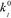Modulation of myosin-actin transitions by the CFC. The probabilities of changing state in the three state model are constructed so that each state can transition to two neighboring states. The transition states from the detached state M.ADP.Pi includes two attachment probabilities       and      associated with axial strain dependent rates, including binding to multiple binding sites on actin, denoted as     . For the crossbridges in the detached state,      , the attachment probability is shared between all reachable actin states defined as                           , where                                                                               and                   . The weight factors        and        are associated with the azimuthal position of actin filaments in the sarcomere lattice relative to the myosin head, angle       , and azimuthal angle of actin site      (see Mijailovich et al., 2016). The weight factors are               are proportional to the fraction of time when these transitions are possible, i.e., to the weighted probabilities that the CFC is at positions             , that modulates weak myosin binding, denoted as subscript                (Mijailovich et al., 2012a).

The transitions from weakly and strongly attached states (       and      ) are defined by probabilities                                      for all transitions from the pre-stroke state into the post stroke state. Calculation of these probabilities is almost identical as reported in (Mijailovich et al., 2016), except            that includes weight factor               that is proportional to the fraction of time when the isomerization possible, i.e.           (Mijailovich et al., 2012a).